Integrating over the Cylinder's Surface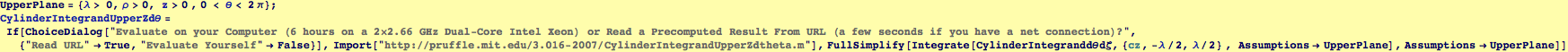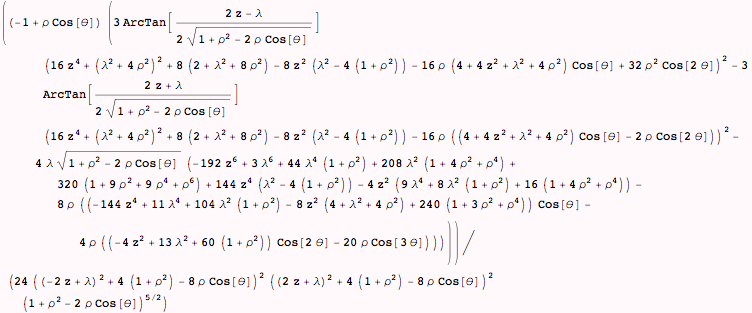Here we restrict z to the upper half-space. We will treat z=0 below.

Here is the limit of  the integral for z> 0 (CylinderIntegranddθ) in the limit as z  → 0.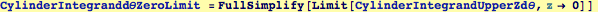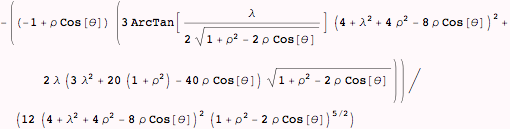Here is the limit of  the integral z=0, it is not obvious that the limit and its value at z=0 are the same. (the following evaluation will take about 10-20 seconds)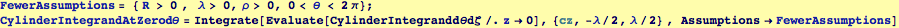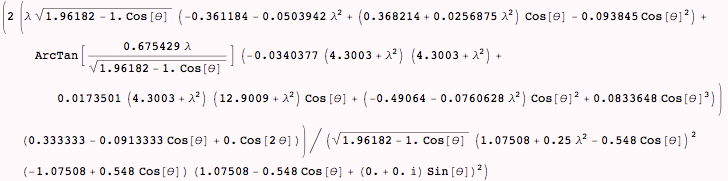The limit as z -> 0 and the integrand at z = 0 are the same, so we can use a single integrand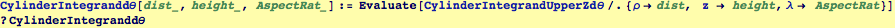Global`CylinderIntegranddθ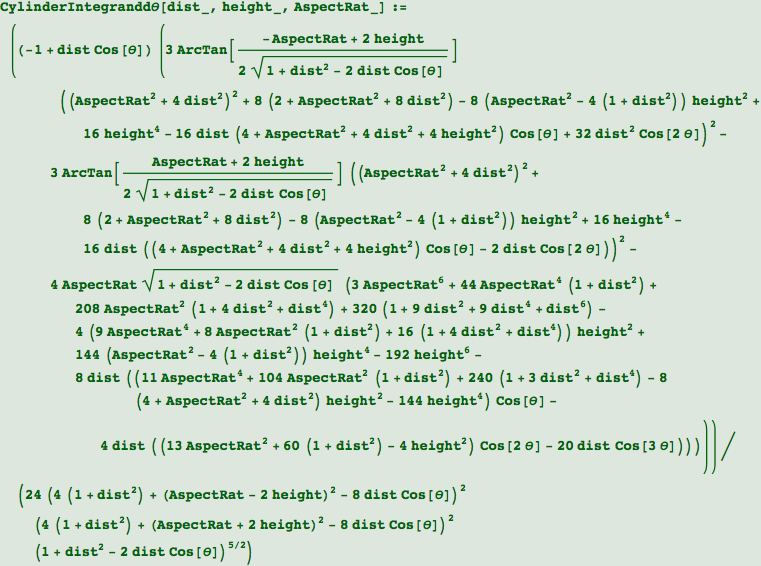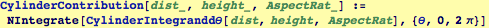Created by Wolfram Mathematica 6.0  (23 October 2007)